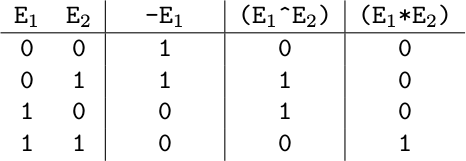Time Limit : sec, Memory Limit : KB

### Boolean Expression Compressor

You are asked to build a compressor for Boolean expressions that transforms expressions to the shortest form keeping their meaning.

The grammar of the Boolean expressions has terminals 0 1 a b c d - ^ * ( ), start symbol <E> and the following production rule:

<E>  ::=  0  |  1  |  a  |  b  |  c  |  d  |  -<E>  |  (<E>^<E>)  |  (<E>*<E>)

Letters a, b, c and d represent Boolean variables that have values of either 0 or 1. Operators are evaluated as shown in the Table below. In other words, - means negation (NOT), ^ means exclusive disjunction (XOR), and * means logical conjunction (AND).

Table: Evaluations of operatorsWrite a program that calculates the length of the shortest expression that evaluates equal to the given expression with whatever values of the four variables.

For example, 0, that is the first expression in the sample input, cannot be shortened further. Therefore the shortest length for this expression is 1.

For another example, (a*(1*b)), the second in the sample input, always evaluates equal to (a*b) and (b*a), which are the shortest. The output for this expression, thus, should be 5.

### Input

The input consists of multiple datasets. A dataset consists of one line, containing an expression conforming to the grammar described above. The length of the expression is less than or equal to 16 characters.

The end of the input is indicated by a line containing one . (period). The number of datasets in the input is at most 200.

### Output

For each dataset, output a single line containing an integer which is the length of the shortest expression that has the same value as the given expression for all combinations of values in the variables.

### Sample Input

0
(a*(1*b))
(1^a)
(-(-a*-b)*a)
(a^(b^(c^d)))
.


### Output for the Sample Input

1
5
2
1
13